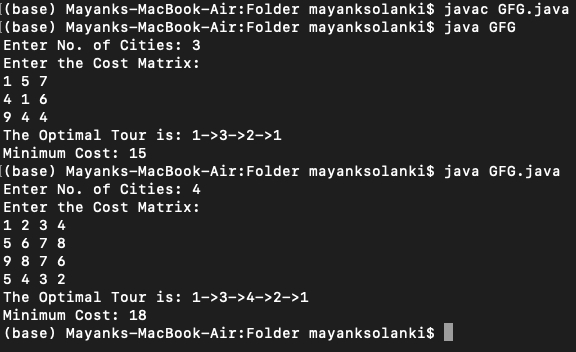GeeksforGeeks App
Open AppBrowser
Continue

# Java Program to Solve Travelling Salesman Problem Using Incremental Insertion Method

Incremental is actually used in software development where the model is designed, implemented, and tested incrementally (a little more is added each time) until the product is finished. It involves both development and maintenance. The product is defined as finished when it satisfies all of its requirements.

Let us briefly discuss the traveling salesman problem. Here we are supposed to find the simplest or shortest distance between all the cities the salesman has to travel i.e., finding the shortest and optimal route between the nodes of the graph. It is also known as TSP and is the most known computer science optimized problem in this modern world.

Algorithm:

2. Find node r such that cir is minimal and form sub-tour i-r-i.
3. (Selection step) Given a sub-tour, find node r not in the sub-tour closest to any node j in the sub-tour; i.e. with minimal crj
4. (Insertion step) Find the arc (i, j) in the sub-tour which minimizes cir + crj – cij Insert ‘r’ between ‘i’ and ‘j’.
5. If all the nodes are added to the tour, stop. Else go to step 3

Implementation:

## Java

 `// Java Program to Solve Travelling Salesman Problem``// Using Incremental Insertion Method` `// Importing input output classes``import` `java.io.*;``// Importing Scanner class to take input from the user``import` `java.util.Scanner;` `// Main class``public` `class` `GFG {``    ``// Method 1``    ``// Travelling Salesman Incremental Insertion Method``    ``static` `int` `tspdp(``int` `c[][], ``int` `tour[], ``int` `start,``                     ``int` `n)``    ``{` `        ``int` `mintour[] = ``new` `int``[``10``], temp[] = ``new` `int``[``10``],``            ``mincost = ``999``, ccost, i, j, k;` `        ``if` `(start == n - ``1``)` `        ``{` `            ``return` `(c[tour[n - ``1``]][tour[n]]``                    ``+ c[tour[n]][``1``]);``        ``}` `        ``// Logic for implementing the minimal cost` `        ``for` `(i = start + ``1``; i <= n; i++)` `        ``{` `            ``for` `(j = ``1``; j <= n; j++)` `                ``temp[j] = tour[j];` `            ``temp[start + ``1``] = tour[i];` `            ``temp[i] = tour[start + ``1``];` `            ``if` `((c[tour[start]][tour[i]]``                 ``+ (ccost = tspdp(c, temp, start + ``1``, n)))``                ``< mincost)` `            ``{` `                ``mincost = c[tour[start]][tour[i]] + ccost;` `                ``for` `(k = ``1``; k <= n; k++)` `                    ``mintour[k] = temp[k];``            ``}``        ``}` `        ``// Now, iterating over the path (mintour) to``        ``// compute its cost``        ``for` `(i = ``1``; i <= n; i++)` `            ``tour[i] = mintour[i];` `        ``// Returning the cost of min path``        ``return` `mincost;``    ``}` `    ``// Method 2``    ``// Main driver method``    ``public` `static` `void` `main(String[] args)` `    ``{``        ``// Creating an object of Scanner class to take user``        ``// input``        ``// 1. Number of cities``        ``// 2. Cost matrix``        ``Scanner in = ``new` `Scanner(System.in);` `        ``// Creating matrices in the main body``        ``int` `c[][] = ``new` `int``[``10``][``10``], tour[] = ``new` `int``[``10``];` `        ``// Declaring variables``        ``int` `i, j, cost;` `        ``// Step 1: To read number of cities` `        ``// Display message for asking user to``        ``// enter number of cities``        ``System.out.print(``"Enter No. of Cities: "``);` `        ``// Reading and storing using nextInt() of Scanner``        ``int` `n = in.nextInt();` `        ``// Base case``        ``// If the city is 1 then``        ``// path is not possible``        ``// Cost doesnot play any role``        ``if` `(n == ``1``) {``            ``// Display on the console``            ``System.out.println(``"Path is not possible!"``);` `            ``// terminate``            ``System.exit(``0``);``        ``}` `        ``// Case 2``        ``// Many cities` `        ``// Again, reading the cost of the matrix` `        ``// Display message``        ``System.out.println(``"Enter the Cost Matrix:"``);` `        ``// Travelling across cities using nested loops``        ``for` `(i = ``1``; i <= n; i++)` `            ``for` `(j = ``1``; j <= n; j++)` `                ``c[i][j] = in.nextInt();` `        ``for` `(i = ``1``; i <= n; i++)` `            ``tour[i] = i;` `        ``// Calling the above Method 1 to``        ``cost = tspdp(c, tour, ``1``, n);` `        ``// Now, coming to logic to print the optimal tour` `        ``// Display message for better readability``        ``System.out.print(``"The Optimal Tour is: "``);` `        ` `        ``for` `(i = ``1``; i <= n; i++)` `            ``// Printing across which cities should Salesman``            ``// travel``            ``System.out.print(tour[i] + ``"->"``);` `        ``// Starting off with the city 1->``        ``System.out.println(``"1"``);` `        ``// Print and display the (minimum)cost of the path``        ``// traversed``        ``System.out.println(``"Minimum Cost: "` `+ cost);``    ``}``}`

Output:My Personal Notes arrow_drop_up